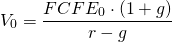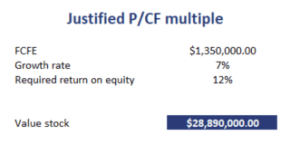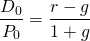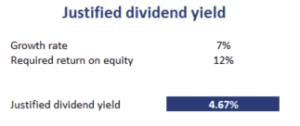## Justified ratios

Justified ratios or justified multiples are multiples based on a company’s fundamentals. Examples include the justified P/E ratio, justified price-to-sales as well as some less well-know ratios such as the justified dividend yield, justified P/CF multiple, and the EV/EBITDA multiple. Using a spreadsheet, we implement some of these multiples. The spreadsheet is available for download below.

## Justified EV/EBITDA multiple

Justified EV/EBITDA multiple is a multiple based on a company’s fundamentals. It is based on the enterprise value (EV) forecasted from fundamentals. The forecasted enterprise value is divided by the Earnings Before Interest, Taxes, Depreciation and Amortization (EBITDA) that is also forecasted from the company’s fundamentals.

The EV/EBITDA multiple is positively related to the growth rate in FCFF and EBITDA. At the same time, the EV/EBITDA multiple is negatively related to the firm’s overall risk level and the WACC.

## Justified P/CF Multiple

The justified P/CF multiple is based on a single stage dividend discount model. In particular, we first calculate the CF measure of interest, and then apply a single stage DCF is illustrated by the formula below. The justified P/CF formula therefore equalswhere FCFE is the Free Cash Flow to Equity, g is the growth rate, and r is the required rate of return on equity. The following table implements a justified P/CF multiple example.## Justified Dividend Yield

Another interesting multiple is the justified dividend yield. It is based on the Gordon Growth Model (GMM). The formula for the justified dividend yield equalsThe justified dividend yield is positively related to the required rate of return and negatively related to the forecasted growth rate in dividends. As a consequence, high dividend yields are associated with value stocks.

The following table illustrates how to apply the formula using Excel. Interestingly, we only need to variables, the growth rate and the required rate of return on equity. The spreadsheet is available below.## Summary

We discussed three popular justified multiples, the justified EV/EBITDA, the justified dividend yield, and the justified price-to-free cash flow. The important thing to remember is that these ratios are based on the company’s fundamentals.

### Justified ratios calculator

Want to have an implementation in Excel? Download the Excel file: Justified Ratios